## Power System Objective questions from competitive questions(GATE, IES)

 Power is transfered from system A to system B by an HVDC link as shown in the figure.If the voltages VAB and VCD are as indicated in the figure, and I>0,thenA. VAB<0,VCD<0,VAB>VCD
B. VAB>0,VCD>0,VAB>VCD
C. VAB>0,VCD>0,VAB<VCD
D. VAB>0,VCD<0

 Consider a step voltage wave of magnitude 1pu travelling along a lossless transmission line that terminates in a reactor.The voltage magnitude across the reactor at the instant the travelling wave reaches the reactor is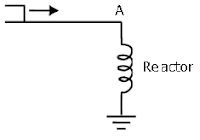A. -1pu
B. 1pu
C. 2pu
D.  3pu

 Consider two buses connected by an impedance of (0+j5)Ω. The bus 1 voltage is 100∠30° V, and bus 2 voltage is 100∠0° V. The real and reactive power supplied by bus 1, respectively are
A. 1000W,268Var
B. -1000W,-134Var
C. 276.9W,-56.7Var
D. -276.9W,56.7Var

 A three-phase, 33kV oil circuit breaker is rated 1200A, 2000MVA, 3s. The symmetrical breaking current is
A. 1200A
B. 3600A
C. 35kA
D. 104.8kA

 Consider a stator winding of an alternator with an internal high-resistance ground fault.The currents under the fault condition are as shown in the figure.The winding is protected using a differential current scheme with current transformers of ratio 400/5A as shown. The current through the operating coil is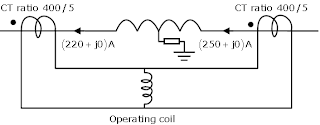A. 0.17875A
B. 0.2A
C. 0.375A
D. 60kA

 A 50Hz synchronous generator is initially connected to a long lossless transmission line which is open circuited at the receiving end.With the field voltage held constant, the generator is disconnected from the transmission line.Which of the following may be said about the steady state terminal voltage and field current of the generator?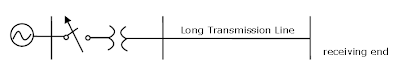A. The magnitude of terminal voltage decreases,and the field current does not change
B. The magnitude of terminal voltage increases,and the field current does not change
C. The magnitude of terminal voltage increases,and the field current increases
D. The magnitude of terminal voltage does not change,and the field current decreases

 Consider a three-phase,50Hz,11kV distribution system.Each of the conductors is suspended by an insulator string having two identical porcelain insulators.The self capacitance of the insulator is 5 times the shunt capacitance between the link and the ground,as shown in the figure.The voltage across the two insulators is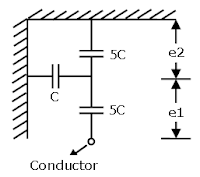A. e1=3.74kV,e2=2.61kV
B. e1=3.46kV,e2=2.89kV
C. e1=6.0kV,e2=4.23kV
D. e1=5.5kV,e2=5.5kV

 Consider a three-core, three-phase,50Hz,11kV cable whose conductors are denoted as R,Y and B in the figure.The inter-phase capacitance(C1) between each pair of conductors is 0.2μF and the capacitance between each line conductor and the sheath is 0.4μF.The per-phase charging current isA. 2.0A
B. 2.4A
C. 2.7A
D. 3.5A

 For the power system shown in the figure below,the specifications of the components are the following:
G1: 25kV,100MVA,X=9%
G2: 25'kV,100MVA,X=9%
T1: 25kV/220kV,90MVA,X=12%
T2: 220kV/25kV,90MVA,X=12%
Line1: 220kV, X=150 ohms.Choose 25kV as the base voltage at the generator G1 and 200MVA as the MVA base.The impedance diagram is.....Options A,B,C,D are given below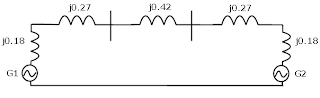For enhancing the power transmission in a long EHV transmission line, the most preferred method is to connect a
A. Series inductive compensator in the line
B. Shunt inductive compensator at the receiving end
C. Series capacitive compensator in the line
D. Shunt capacitive compensator at the sending end

2:26 PM

#### 1 comment:

1.Question no:1 answer should be B please check.
Reason:current flows from high voltage to low voltage